Next: iclass.06 Up: Invited Classroom Day Presentations Previous: iclass.04

# iclass.05

CLASSROOM DAY

Session Slot: Monday, 2:00 - 2:55

AudioVisual Request: None

Session Organizer: Scott, David W.

Session Title: Classroom Day

1. Quotient Spaces in Statistical Models

McCullagh, Peter,   University of Chicago

Address: Department of Statistics University of Chicago 5734 University Ave Chicago, IL 60637

Phone: 773-702-8340

Fax: 773-702-9810

Email: pmcc@roxbee.uchicago.edu

Abstract: A statistical variable, or variate for short, is a function, usually real-valued, on the statistical units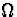.Each variate is thus an element in the vector space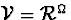.Many statistical models, including all linear models and generalized linear models, are specified in terms of subspaces, usually in the form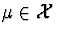,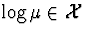, or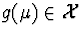, where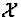is the span of the model matrix X. Quotient spaces are rarely considered explicitly in statistical work, but it will be argued in this talk that this oversight is a mistake. Quotient spaces do arise naturally in various settings of which the following are typical examples.

In the case of the linear model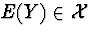,the residual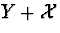lies in the quotient space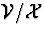.An elementary calculation shows thatis normally distributed in the quotient space, whatever this may mean, and the likelihood function based onis the REML likelihood.

In testing a composite null hypothesis H0 against an alternative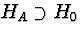,if both both hypotheses correspond to vector subspaces, the standard test statistics are based on the quotient space projection on to HA/H0.

Interaction between two factors A, B, is the quotient-space projection Y+(A+B) of the response Y on to the space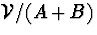,i.e. Y modulo additivity. Regardless of whether the design is balanced, the interaction sum of squares is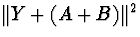, or more generally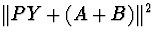, where P is the orthogonal projection on to A.B.

In the case of multinomial response models, the probability vector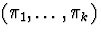is a point in the probability simplex in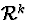.The usual link transformation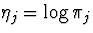has inverse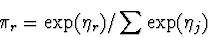so that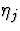and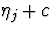are equivalent points. In other words,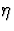is a point in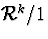, and the logistic link is 1-1 from the interior of the simplex onto.

Bayes's theorem is quotient-space vector addition.

For incomplete data, if the relation between the incomplete response and the complete response is linear, the incomplete response is most naturally viewed as a point in a certain quotient space. Although more restrictive than the EM algorithm, this formulation leads to a simple theory of estimation using estimating functions.

The lecture will be in the form of an expository review session, beginning with the definition of a quotient space, continuing with inner products norms and Lebesgue measure on quotient spaces, and deriving the normal distribution on.Details of this and other examples listed above can be found at http://galton.uchicago.edu/~pmcc/quotient.ps

KeyWords: interaction, analysis of variance, multinomial response model, REML

AudioVisual Request: NoneNext: iclass.06 Up: Invited Classroom Day Presentations Previous: iclass.04
David Scott
6/1/1998